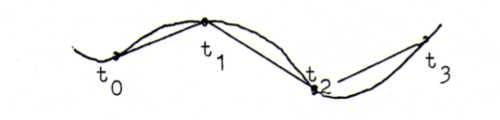### DIFFERENTIAL GEOMETRY COURSE

2. ARC LENGTH

Definition 10: A curve is the range of a mapping I→ℜ3, where I is an interval on the real axis. We always silently suppose that the mapping is as often continuously differentiable as is necessary in order to have our assertions hold.
We denote the image of t as x(t) = (x1(t),x2(t),x3(t)).
Note that distinct mappings distinct parametrisations) may define the same curve. For example, the circle is given by (cos(t),sin(t),0) and by (sin(2t),cos(2t),0).

Example 11:
1) straight line: x(t) = s + t v;
2) circular helix: x(t) = (a.cos(t),a.sin(t),bt), intersection of a circular cylinder x12+x22=a2 and a wave plate cylinder x2=a.sin(x3/b);
3) cubic parabola: x(t) = (t, t2, t3), intersection of a parabolical cylinder x2=x12 and the cubic cylinder x3=x13.

Definition 12: a singular point of a curve with parametrisation x(t) is a point x(t0) where x'(t0)=0. A point that is not singular is called regular. See also problem 13.
Suppose that x(t1) is a regular point. Then x(t) = x(t1) + (t-t1)x'(t1) + O((t-t1)2). We call the straight line with parametrisation x(t1) + λx'(t1) tangent in x(t1) to the curve x(t), and the direction vector x'(t1) tangent vector.
We can also explain this in a geometrical way:The connecting line has direction vector (x(t1+Δt)-x(t1))/Δt.
When Δt→0, the connecting line becomes the tangent and the direction vector becomes x'(t1).

Problem 13: Substitute t=u3 in example 11 3). Show that we get the same curve, but that with this parametrisation it has a singular point.

Problem 14: In the examples 11 2) and 11 3), determine the point of intersection of the tangent in x(t1) and the plane x1=0. For which t1 is this intersection point a point at infinity?

Definition 15: We approximate the length along the curve x(t) between t0 and t by taking between t0 and t a number of points tk and calculating Σ ||x(tk+1)-x(tk)||.This sum is equal to Σ ||x'(τk)(tk+1-tk)||, where τk lies between tk and tk+1.
When we take the limit we get the following definition of the arc length from x(t0) to x(t):
s(t) = t0t ||x'(τ)|| dτ = t0t √(x1'(τ)2+x2'(τ)2+x3'(τ)2) dτ.

According to the pricipal proposition of the integral calculus we find ds/dt = ||x'(τ)||, and hence ||d/ds x(s)|| = ||x'(t)|| dt/ds = 1.

Henceforth we will write x .(s) instead of d/ds x(s), so we show by the little dot that we mean the derivative with respect to arc length.

According to definition 12, the tangent in x(s1) is x(s1)+λx .(s1), where the tangent vector x .(s1) has length 1.

Problem 16: Give a parametrisation of the circular helix with arc length as parameter. Give the tangent vector, too.

Problem 17: Give a parametrisation of the planar curve y=x3/2, with arc length as parameter.

Problem 18: Calculate the arc length of the cycloid a(t-sin(t),1-cos(t)), and draw this curve.
Show that it gives the traject of a point P on a circle rolling over a straight line.

Problem19: Determine f(t) such that angle between the curve x(t) = f(t)(cos(t),sin(t),1) on the cone x12+x22=x32 and the straight lines on this cone is the same in all points of intersection.

Problem 20: A curve is given by two parametrisations with parameters t and s respectively, so that x(s) = x(t(s)) and x(t) = x(s(t)).
Let x'' be the second derivative with respect to t, and x .. the second derivative with respect to s.
Express ||x ..|| in x' and x''.

(Answer ||x ..|| = ||x'⊗x''||/||x'||3. This answer is important for the calculation of the curvature, which we will describe in the next section.)